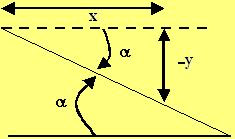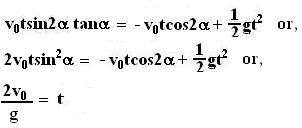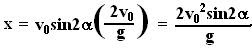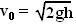## Wednesday, July 18, 2007

### Irodov Problem 1.31The entire event is depicted in the figure. The ball first falls from a height h on to the inclined plane and then reflects off of the plane. The ball will hit the plane at an angleand thus will at an angle that forms an equal anglewith the normal to the plane. Thus, the ball will reflect off of the inclined plane at an angle ofwith the horizontal as shown in the figure.

Let the point of contact of the ball with the inclined plane for the first time be the origin in our Cartesian coordinate system and let t=0. Then the position of the ball after reflection at any time is given by,Here V0 is the velocity at first impact on the inclined plane.Now this ball must meet the inclined plane. Any point on the inclined plane satisfies the relationThis can be seen from the figure drawn beside.

Thus where ever the ball meets the plane, the inclined plane relation must also hold true. In other words,Now knowing the time we can calculate the x coordinate of where the ball hits the inclined plane as,Now the distance l traveled by the ball along the inclined plane is given as,Since the ball fell a height h before hitting the inclined plane, it must have attained a velocity of. This relation can be obtained by either equating the potential energy lost by the balls (mgh) during the fall and equating to its kinetic energy upon impact (½ mv02) . Now substituting this value of initial velocity in to our found value of l we have,#### 1 comment:Anonymous said...

Solution is slightly more elegant if you rotate the frame by an angle theta so that the inclined plane becomes horizontal. This can be treated like the standard object thrown at theta except that the horizontal direction also has an acceleration.